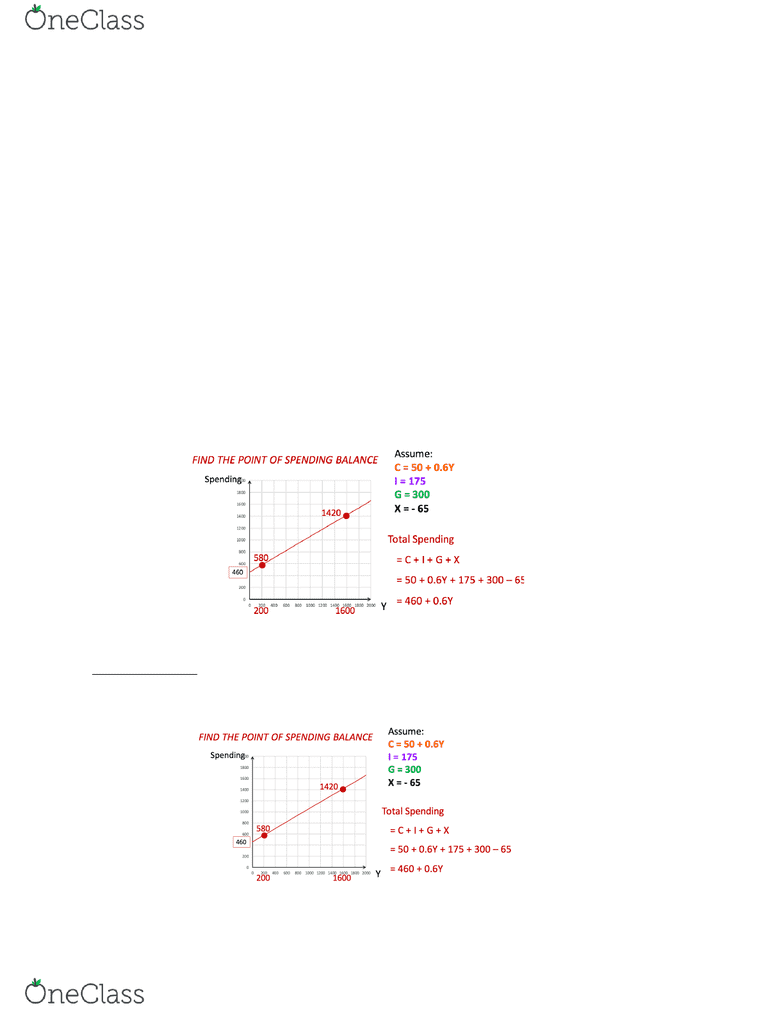# ECON-002 Lecture Notes - Lecture 23: Fiscal Multiplier, Aggregate Demand

56 views3 pages
School
Department
Course
ProfessorApril 20 Lecture
The Spending Balance Model!
Simple Version. Assume
C: depends on Y
I: does not depend on Y (+)
G: does not depend on Y
X: does not depend on Y (-)
I, G, and X are together referred to as autonomous spending (i.e. they don’t depend on Y)
Spending Balance if Y = C + I + G + X
Is this not always the case? C, I, G, and X are desired shares, not actual shares. Investment is particularly
problematic, particularly when business investment is not desired. EX: in a recession, firms have trouble
selling goods, goods accumulate as inventory. Inventory counts as investment, but it is not desired
investment.
Graph of the Spending Balance Model starts with the expenditure line. This line graphs (also referred to
as expenditure line) plots desired spending as a function of Y.
In the example in class, Professor Rogers graphs a spending curve with the equation Spending=460+0.6Y
Where is the point of spending balance?
GRAPHICAL HACK: You can guess and check to find the point where Spending=Y (not efficient)
Graphical method: draw a 45 degree line on the graph (line from the origin to the top right corner). At
every point along the 45 degree line, Spending = Y. The point of Spending balance is the point where the
expenditure line intersects the 45 degree line.
find more resources at oneclass.com
find more resources at oneclass.com
Unlock document

This preview shows page 1 of the document.
Unlock all 3 pages and 3 million more documents.